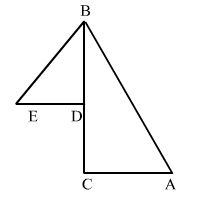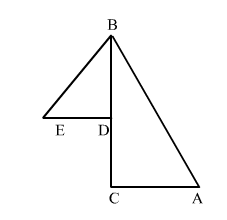# In the given figure, D is a point on side BC of a ΔABC and E is a point such that CD = DE. Prove that AB + AC > BE.

Question:

In the given figure, D is a point on side BC of a ΔABC and E is a point such that CD DE. Prove that AB AC BE.Solution:Given: CD DE

To prove: AB AC BE

Proof:

In $\Delta A B C$

$A B+A C>B C \quad \ldots(1)$

In $\Delta B E D$

$B D+C D>B E$

$\Rightarrow B C>B E \quad \ldots(2)$

From (1) and (2), we get

$A B+A C>B E$